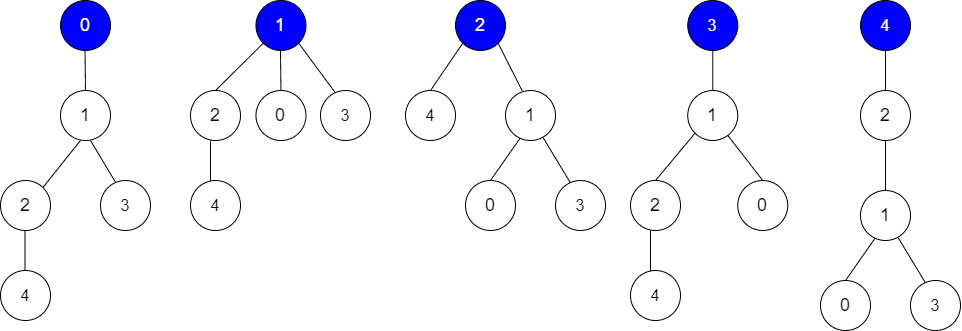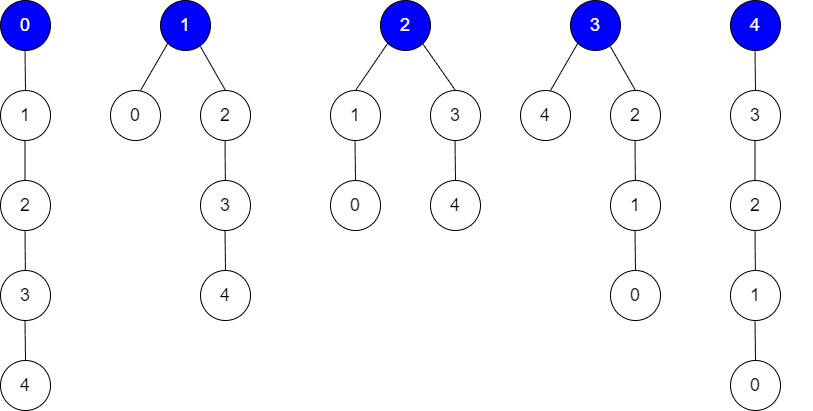##### Welcome to Subscribe On Youtube

Formatted question description: https://leetcode.ca/all/2581.html

# 2581. Count Number of Possible Root Nodes

## Description

Alice has an undirected tree with n nodes labeled from 0 to n - 1. The tree is represented as a 2D integer array edges of length n - 1 where edges[i] = [ai, bi] indicates that there is an edge between nodes ai and bi in the tree.

Alice wants Bob to find the root of the tree. She allows Bob to make several guesses about her tree. In one guess, he does the following:

• Chooses two distinct integers u and v such that there exists an edge [u, v] in the tree.
• He tells Alice that u is the parent of v in the tree.

Bob's guesses are represented by a 2D integer array guesses where guesses[j] = [uj, vj] indicates Bob guessed uj to be the parent of vj.

Alice being lazy, does not reply to each of Bob's guesses, but just says that at least k of his guesses are true.

Given the 2D integer arrays edges, guesses and the integer k, return the number of possible nodes that can be the root of Alice's tree. If there is no such tree, return 0.

Example 1:Input: edges = [[0,1],[1,2],[1,3],[4,2]], guesses = [[1,3],[0,1],[1,0],[2,4]], k = 3
Output: 3
Explanation:
Root = 0, correct guesses = [1,3], [0,1], [2,4]
Root = 1, correct guesses = [1,3], [1,0], [2,4]
Root = 2, correct guesses = [1,3], [1,0], [2,4]
Root = 3, correct guesses = [1,0], [2,4]
Root = 4, correct guesses = [1,3], [1,0]
Considering 0, 1, or 2 as root node leads to 3 correct guesses.



Example 2:Input: edges = [[0,1],[1,2],[2,3],[3,4]], guesses = [[1,0],[3,4],[2,1],[3,2]], k = 1
Output: 5
Explanation:
Root = 0, correct guesses = [3,4]
Root = 1, correct guesses = [1,0], [3,4]
Root = 2, correct guesses = [1,0], [2,1], [3,4]
Root = 3, correct guesses = [1,0], [2,1], [3,2], [3,4]
Root = 4, correct guesses = [1,0], [2,1], [3,2]
Considering any node as root will give at least 1 correct guess.



Constraints:

• edges.length == n - 1
• 2 <= n <= 105
• 1 <= guesses.length <= 105
• 0 <= ai, bi, uj, vj <= n - 1
• ai != bi
• uj != vj
• edges represents a valid tree.
• guesses[j] is an edge of the tree.
• guesses is unique.
• 0 <= k <= guesses.length

## Solutions

• class Solution {
private List<Integer>[] g;
private Map<Long, Integer> gs = new HashMap<>();
private int ans;
private int k;
private int cnt;
private int n;

public int rootCount(int[][] edges, int[][] guesses, int k) {
this.k = k;
n = edges.length + 1;
g = new List[n];
Arrays.setAll(g, e -> new ArrayList<>());
for (var e : edges) {
int a = e, b = e;
}
for (var e : guesses) {
int a = e, b = e;
gs.merge(f(a, b), 1, Integer::sum);
}
dfs1(0, -1);
dfs2(0, -1);
return ans;
}

private void dfs1(int i, int fa) {
for (int j : g[i]) {
if (j != fa) {
cnt += gs.getOrDefault(f(i, j), 0);
dfs1(j, i);
}
}
}

private void dfs2(int i, int fa) {
ans += cnt >= k ? 1 : 0;
for (int j : g[i]) {
if (j != fa) {
int a = gs.getOrDefault(f(i, j), 0);
int b = gs.getOrDefault(f(j, i), 0);
cnt -= a;
cnt += b;
dfs2(j, i);
cnt -= b;
cnt += a;
}
}
}

private long f(int i, int j) {
return 1L * i * n + j;
}
}

• class Solution {
public:
int rootCount(vector<vector<int>>& edges, vector<vector<int>>& guesses, int k) {
int n = edges.size() + 1;
vector<vector<int>> g(n);
unordered_map<long long, int> gs;
auto f = [&](int i, int j) {
return 1LL * i * n + j;
};
for (auto& e : edges) {
int a = e, b = e;
g[a].push_back(b);
g[b].push_back(a);
}
for (auto& e : guesses) {
int a = e, b = e;
gs[f(a, b)]++;
}
int ans = 0;
int cnt = 0;

function<void(int, int)> dfs1 = [&](int i, int fa) {
for (int& j : g[i]) {
if (j != fa) {
cnt += gs[f(i, j)];
dfs1(j, i);
}
}
};

function<void(int, int)> dfs2 = [&](int i, int fa) {
ans += cnt >= k;
for (int& j : g[i]) {
if (j != fa) {
int a = gs[f(i, j)];
int b = gs[f(j, i)];
cnt -= a;
cnt += b;
dfs2(j, i);
cnt -= b;
cnt += a;
}
}
};
dfs1(0, -1);
dfs2(0, -1);
return ans;
}
};

• class Solution:
def rootCount(
self, edges: List[List[int]], guesses: List[List[int]], k: int
) -> int:
def dfs1(i, fa):
nonlocal cnt
for j in g[i]:
if j != fa:
cnt += gs[(i, j)]
dfs1(j, i)

def dfs2(i, fa):
nonlocal ans, cnt
ans += cnt >= k
for j in g[i]:
if j != fa:
cnt -= gs[(i, j)]
cnt += gs[(j, i)]
dfs2(j, i)
cnt -= gs[(j, i)]
cnt += gs[(i, j)]

g = defaultdict(list)
for a, b in edges:
g[a].append(b)
g[b].append(a)
gs = Counter((u, v) for u, v in guesses)
cnt = 0
dfs1(0, -1)
ans = 0
dfs2(0, -1)
return ans


• func rootCount(edges [][]int, guesses [][]int, k int) (ans int) {
n := len(edges) + 1
g := make([][]int, n)
gs := map[int]int{}
for _, e := range edges {
a, b := e, e
g[a] = append(g[a], b)
g[b] = append(g[b], a)
}
f := func(i, j int) int {
return i*n + j
}
for _, e := range guesses {
a, b := e, e
gs[f(a, b)]++
}

cnt := 0
var dfs1 func(i, fa int)
var dfs2 func(i, fa int)
dfs1 = func(i, fa int) {
for _, j := range g[i] {
if j != fa {
cnt += gs[f(i, j)]
dfs1(j, i)
}
}
}
dfs2 = func(i, fa int) {
if cnt >= k {
ans++
}
for _, j := range g[i] {
if j != fa {
a, b := gs[f(i, j)], gs[f(j, i)]
cnt -= a
cnt += b
dfs2(j, i)
cnt -= b
cnt += a
}
}
}
dfs1(0, -1)
dfs2(0, -1)
return
}